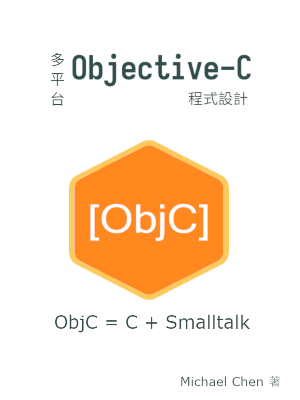# 控制物件的存取權限以實現封裝

## 前言

在先前的範例程式中，我們不強調屬性和訊息的存取權限。也就是說，在預設情形下，類別使用者看得到該類別的屬性，所有的訊息都是公開的。但我們有時候想要隱藏一部分屬性和訊息，僅保持最小量的公開界面。本文展示在 Objective-C 類別中實作私有屬性和私有訊息的方式。

## 陣列類別 `Array` 的公開界面宣告

我們先來看此陣列的公開界面：

``````/* array.h */
#pragma once

#import <Foundation/Foundation.h>
#include <stdbool.h>
#include <stddef.h>

typedef struct array_data_t array_data_t;

@interface Array : NSObject {
array_data_t *data;
}

-(Array *) init;
-(void) dealloc;
-(size_t) size;
-(id) at: (size_t) index;
-(bool) push: (id) obj;
@end
``````

類別 `Array` 的屬性 `data` 表面上是公開的，但 `data` 本身是 opaque pointer，對類別 `Array` 的使用者來說，其屬性 `data` 實質上是隱藏的。另外，我們也實作了一個私有訊息，但從公開界面中無法看到該訊息。

## 陣列類別 `Array` 的內部實作

接著，我們來看該類別內部的實作。

我們先看私有屬性的部分：

``````struct array_data_t {
size_t size;
size_t capacity;
size_t tail;
id *elems;
};
``````

我們用結構體 `array_data_t` 把真正的屬性包在裡面。由於該結構體放在實作檔中，對外部程式來說，無法直接存取此結構體內的屬性，故類別 `Array` 的屬性視為私有。

另外，我們來看私有訊息的部分：

``````@interface Array ()
-(bool) expand;
@end
``````

在這裡，我們用到了 Objective-C 的語法特性 category。原本 category 的用意是用來延伸現存的類別，我們把 `Array` 的 category 界面藏在實作檔中，對於類別使用者來說同樣視為不可見。

由於 Objective-C 是動態語言，其實類別使用者仍然可以呼叫這個訊息。但該訊息對類別使用者來說是不可見的，類別使用者若硬要呼叫該訊息時，編譯器會發出警告訊息。

我們來看如何初始化此物件：

``````-(Array *) init
{
if (!self)
return self;

self = [super init];

data = \
(array_data_t *) malloc(sizeof(array_data_t));
if (!data) {
[self release];
self = nil;
return self;
}

data->size = 0;
data->capacity = 2;
data->tail = 0;

data->elems = \
(id *) malloc(data->capacity * sizeof(id));
if (!(data->elems)) {
free(data);
[self release];
self = nil;
return self;
}

return self;
}
``````

我們在內部偷偷地使用標準 C 的 `malloc()` 函式來配置記憶體，因為 `data` 本質上是指向結構體的指標，所以無法用 Objective-C 提供的 `alloc` 訊息來配置記憶體。在 Objective-C 程式碼中混用純 C 程式碼是合法的，只要寫得正確即可。

由於我們使用到 `malloc()` 函式，我們在 `dealloc` 訊息中也要自己寫上相對應的 `free()` 函式，不能完全依賴 Objective-C 現成的 `dealloc` 訊息。我們的 `dealloc` 訊息的實作如下：

``````-(void) dealloc
{
if (!self)
return;

if (!data) {
[super dealloc];
return;
}

for (size_t i = 0; i < data->size; ++i) {
size_t index = (i + data->head) % data->size;
if (nil != data->elems[index])
[data->elems[index] release];
}

free(data->elems);
free(data);

[super dealloc];
}
``````

在這個 `dealloc` 訊息中，使用 `malloc()` 所配置的記憶體，得自行用 `free()` 釋放掉。其他的部分則使用 Objective-C 現有的 `dealloc` 訊息來釋放記憶體即可。如同純 C 程式，要由內部向外部逐一釋放，以免因 dangling pointer 無法正確釋放記憶體。

接著，我們來看如何把物件推入此陣列：

``````-(bool) push: (id)obj
{
if (![self expand])
return false;

if (data->size > 0)
data->tail = (data->tail + 1) % data->capacity;

data->elems[data->tail] = obj;
data->size += 1;

return true;
}
``````

一開始，要先確認動態陣列的容量是充足的，所以我們用 `[self expand]` 預擴展好足夠的空間。`expand` 對於此範例程式來說算是私有訊息，因為 `Array` 的公開界面中沒有宣告此訊息。

當陣列大小不為零時，我們得把 `data->tail` 以環狀陣列的手法平移 `1`。最後將 `obj` 放入陣列即可。

剩下的部分基本上和存取權限無關，單純是資料結構方面的議題，我們就不逐一說明。讀者可試著自行閱讀我們提供的範例程式碼：

``````/* array.m */
#include <stdbool.h>
#include <stdlib.h>
#import "array.h"

/* Private fields. */
struct array_data_t {
size_t size;
size_t capacity;
size_t tail;
id *elems;
};

/* Private messages. */
@interface Array ()
- (bool) expand;
@end

@implementation Array
-(Array *) init
{
if (!self)
return self;

self = [super init];

data = (array_data_t *) malloc(sizeof(array_data_t));
if (!data) {
[self release];
self = nil;
return self;
}

data->size = 0;
data->capacity = 2;
data->tail = 0;

data->elems = (id *) malloc(data->capacity * sizeof(id));
if (!(data->elems)) {
free(data);
[self release];
self = nil;
return self;
}

for (size_t i = 0; i < data->capacity; ++i)
data->elems[i] = NULL;

return self;
}

-(void) dealloc
{
if (!self)
return;

if (!data) {
[super dealloc];
return;
}

for (size_t i = 0; i < data->size; ++i) {
size_t index = (i + data->head) % data->size;
if (nil != data->elems[index])
[data->elems[index] release];
}

free(data->elems);
free(data);

[super dealloc];
}

-(size_t) size
{
return data->size;
}

-(id) at: (size_t)index
{
NSAssert(index < data->size, @"Invalid index\n");

size_t i = (index + data->head) % data->size;

return data->elems[i];
}

-(bool) push: (id) obj
{
if (![self expand])
return false;

if (data->size > 0)
data->tail = (data->tail + 1) % data->capacity;

data->elems[data->tail] = obj;
data->size += 1;

return true;
}

-(bool) expand
{
if (data->size < data->capacity)
return true;

data->capacity <<= 1;
id *old_elems = data->elems;
id *new_elems = (id *) malloc(data->capacity * sizeof(id));
if (!new_elems)
return false;

size_t j = 0;
size_t sz = 0;
while (sz < data->size) {
new_elems[j] = old_elems[i];

i = (i + 1) % data->size;
j = (j + 1) % data->capacity;
sz++;
}

data->tail = data->size - 1;

data->elems = new_elems;
free(old_elems);

return true;
}
@end
``````

## 使用該類別的外部程式

最後，我們藉由一個簡短的外部程式來看 `Array` 類別如何使用：

``````/* main.m */
#import <Foundation/Foundation.h>
#include <stdio.h>
#import "array.h"

int main(void)
{
NSAutoreleasePool *pool = \
[[NSAutoreleasePool alloc] init];
if (!pool)
return 1;

Array *arr = nil;

arr = [[[Array alloc] init] autorelease];
if (!arr) {
perror("Failed to allocate arr\n");
goto ERROR;
}

NSArray *data = [NSArray arrayWithObjects:
[NSNumber numberWithInt: 3],
[NSNumber numberWithInt: 4],
[NSNumber numberWithInt: 5],
[NSNumber numberWithInt: 6],
[NSNumber numberWithInt: 7],
nil
];
for (id obj in data) {
if (![arr push: obj]) {
perror("Failed to push data\n");
goto ERROR;
}
}

for (size_t i = 0; i < [arr size]; i++) {
int a = [[arr at:i] intValue];
int b = [[data objectAtIndex: i] intValue];
if (!(a == b)) {
fprintf(stderr, "Unequal value: %d %d\n", a, b);
goto ERROR;
}
}

[pool drain];

return 0;

ERROR:
[pool drain];

return 1;
}
``````

一開始，我們建立 `Array` 物件 `arr`。然後，我們用陣列實字建立 `NSArray` 物件 `data`。我們利用 `for` 迴圈把 `data` 內的資料逐一推入 `arr` 中。最後再用另一個 `for` 迴圈逐一確認兩邊的資料相等。

我們的範例程式所做的事很簡單，只是加上錯誤處理的程式碼，所以看起來稍長一些。

## 編譯此範例程式

在 Mac 上用 Clang 編譯此程式時，不需指定 Cocoa 的路徑，所以指令較為簡單：

``````\$ clang -o array array.m main.m -lobjc -framework Foundation
``````

在類 Unix 系統上用 GCC 編譯此指令時，由於 GNUstep 通常不位於標準路徑，所以要加入 GNUstep 位置相關的參數：

``````\$ gcc -std=c11  -o array array.m main.m -lobjc -lgnustep-base -I /usr/include/GNUstep -L /usr/lib/GNUstep -fconstant-string-class=NSConstantString
``````

在類 Unix 系統上使用 Clang 時也是相同的情形。此外，還要引入 Objective-C 低階物件標頭檔相關的路徑：

``````\$ clang -std=c11 -o arrayDemo array.m main.m -lobjc -lgnustep-base -I /usr/include/GNUstep -I /usr/lib/gcc/x86_64-linux-gnu/7/include -L /usr/lib/GNUstep -fconstant-string-class=NSConstantString
``````

## 結語

實作私有屬性和私有訊息背後的意圖就是實現封裝 (encapsulation)。對於類別來說，我們應該只開放最小足量的訊息，並隱藏所有的屬性，以免外部程式過度依賴類別的內部實作，造成日後修改程式碼的困難。

雖然封裝不是物件必需的條件，良好的封裝的確可以增進程式碼的品質。如果讀者想封裝 Objective-C 物件，可以參考本範例程式的手法。

## 電子書籍

如果你覺得這篇 Objective-C 的技術文章對你有幫助，可以看看這本 Objective-C 程式設計電子書：【分享本文】